# Family

Dad has two years more than mom. Mom has 5 times more than Katy. Katy has 2 times less than Jan. Jan is 10 years old. How old is everyone in the family? How old are all together?

Correct result:

J =  10
K =  5
M =  25
O =  27
s =  67

#### Solution:

$K=10\mathrm{/}2=5$
$O=2+25=27$
$s=27+25+5+10=67$We would be pleased if you find an error in the word problem, spelling mistakes, or inaccuracies and send it to us. Thank you!## Next similar math problems:

• Dividing by five and tenNumber 5040 divide by the number 5 and by number 10: a = 5040: 5 b = 5040: 10
• Multiples of int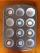How many multiples of 3 is between the numbers to 20? (zero not count)
• Flour2 kg of flour costs 100 CZK. How much does it cost half a kilogram?
• What isWhat is the value of the smaller of a pair of numbers for which their sum is 78 and their division quotients are 0.3?
• Skiing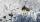Ski gondola starts at a distance of 975 m from the top and is 389 meters long. I went to the bottom station to the top station. How many meters I must go to the top of hill?
• Salary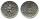Mr. Vesely got for work 874 CZK (Czech Republic Koruna). Mr. Jaros got twice less than Mr. Vesely. How much CZK got Mr. Jaros?
• Fishing boat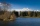The fishing boat caught 14 fish in one day. How many fish will catch 4 fishing boats in 8 days?
• BreederSeven hens breeder has supplied 350 eggs. How long can deliver, if each of its chickens can withstand at least 5 eggs for week?
• Number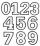Which number is 17 times larger than the number 6?
• Two numbersFind the four times smaller number for numbers 12 and 36 and then add them.
• The machineThe machine works 7 hours a day and produces 1 part in 5 minutes. How many parts will it produce in 1 hour? How many parts will it produce in 1 day?
• Bakers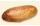Baker Martin baked 5 times more cakes than Dennis. How many cakes baked Dennis if Martin bake 25 cakes?
• MultiplesFind all multiples of 10 that are larger than 136 and smaller than 214.
• Fruit candies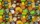Zuzka and Janka ate fruit candies. Zuzka ate 10 and Janka ate 3 times more. How many sweets did both girls eat together?
• Each chair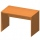Each chair costs twice less than two tables. One table costs 720 Czech crowns. How many crowns are the table and 4 chairs?
• Saving moneyMarko saved € 64. Milan saved 8 euros less than Marko. Marika saved 6 times more than Milan. Calculate who saved how much.
• Stamps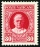Tibor has four times more stamps than Miro and seven times more stamps than Stano. How many stamps have all three if Tibor has 504 stamps?